Keyah Math Module 12, Level 4

Mathematical Content: Exponential and logarithmic functions, algebraic operations, graphs

# Finding the Age of the Earth: Level 4

Solution to Question 1.

Use the basic decay equation P(t) = P0e- λ t. When
t = half-life for Rb-Sr = 4.88 x 104 million years,
then the initial number of atoms is reduced by half (from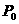to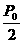) , so denoting half-life by h, set t = h and solve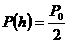for l.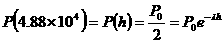,

So now we solve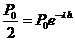for λ, with h = 4.88 x 104.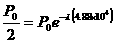Cancel P0 from each side, then take the natural logarithm of both sides,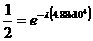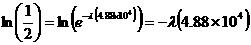But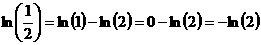so.

We have found the decay constant λ = 1.42 x10-5 for the Rb-Sr system.

This material is based upon work supported by the National Science Foundation under Grant GEO-0355224. Any opinions, findings, and conclusions or recommendations expressed in this material are those of the authors and do not necessarily reflect the views of the National Science Foundation.